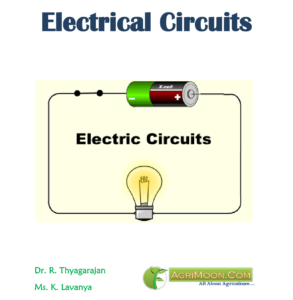You are here

# Book Detail: Electrical Circuits

Language: EnglishPages: 180

Author: Dr. R. Thyagarajan, Ms. K. Lavanya

Price: Free

## Outlines of Electrical Circuits

Module 1. Average and effective value of sinusoidal and linear periodic wave forms

LESSON 1. Average and effective value of sinusoidal forms

LESSON 2. Linear periodic wave forms

Module 2. Independent and dependent sources, loop current and loop equations (Mesh current method)

LESSON 3. Independent Sources

LESSON 4. Dependent sources

LESSON 5. Loop current and loop equations (Mesh current method)

Module 3. Node voltage and node equations (Nodal voltage method)

LESSON 6. Node voltage and node equation (Nodal voltage method)

LESSON 7. Node Analysis

Module 4. Network theorems Thevenin’ s, Norton’ s, Superposition

LESSON 8. Network theorems: Superposition

LESSON 9. Network theorems Thevenin’s and Norton’s

Module 5. Reciprocity and Maximum power transfer

LESSON 10. Maximum Power Transfer Theorem

LESSON 11. Reciprocity Theorem

Module 6. Star- Delta conversion solution of DC circuit by Network theorems

LESSON 12. Star- Delta conversion solution of DC circuit by Network theorems

LESSON 13. Delta to Star Transformations solution of DC circuit by Network theorems

Module 7. Sinusoidal steady state response of circuits

LESSON 14. Sinusoidal steady state response of circuit

LESSON 15. Sinusoidal Response of R-L & R-C Circuit

LESSON 16. Sinusoidal Response of R-L -C Circuit

Module 8. Instantaneous and average power, power factor, reactive and apparent power

LESSON 17. Instantaneous and average power

LESSON 18. Power factorand apparent power

LESSON 19. Reactive power and power triangle

Module 9. Concept and analysis of balanced polyphase circuits

LESSON 20. Polyphase System

LESSON 21. Voltage, Current and Power in a Star and Delta Connected System

LESSON 22. Three-Phase Balanced Circuits

Module 10. Laplace transform method of finding step response of DC circuits

LESSON 23. Laplace Transform method of finding step response of DC circuits

LESSON 24. Functional and operational Transforms

LESSON 25. Laplace Transform of Periodic Functions and inverse transforms

Module 11. Series and parallel resonance

LESSON 26. Series Resonance

LESSON 27. Parallel Resonance

Module 12. Classification of filters

LESSON 28. Classification of filters

LESSON 29. Classification of pass Band and Stop Band

Module 13. Constant-k, m-derived, terminating half network and composite filters

LESSON 30. Constant-k filters

LESSON 31. m-derived filters

LESSON 32. Terminating half network and composite filters

For Daily Update follow us at:

Disclaimer: The information on this website does not warrant or assume any legal liability or responsibility for the accuracy, completeness or usefulness of the courseware contents.

The contents are provided free for noncommercial purpose such as teaching, training, research, extension and self learning.